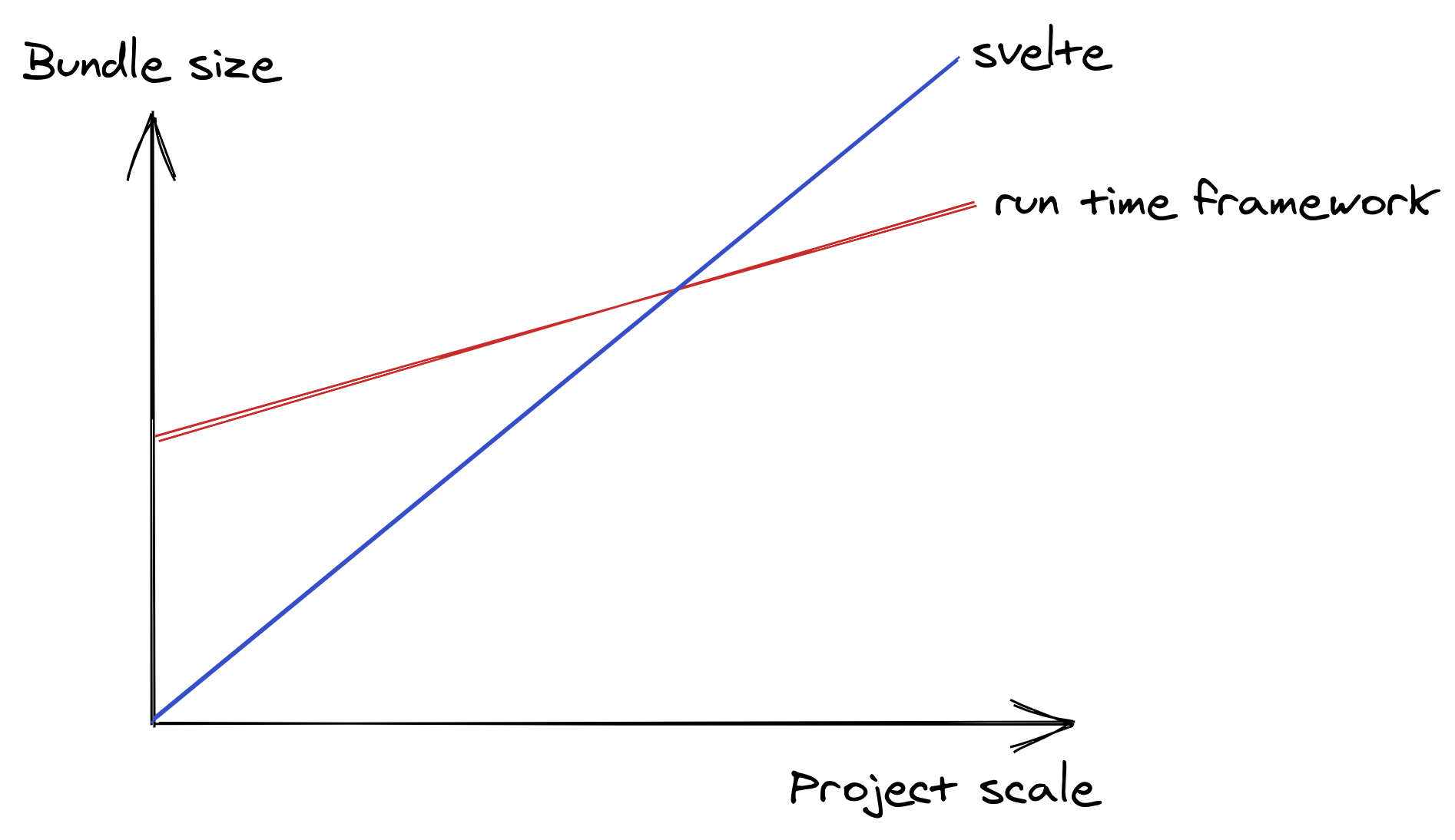# 我对 Svelte 的看法

2021/03/09

## Svelte 是什么？

``````let a = 1
let b = 2
let c = a + b
console.log(c) //=> 3
a = 2
console.log(c) //=> 3, not 4``````

JavaScript 没有 Reactivity 的能力，所以即使 `let c = a + b`, 在改变了 `a``b` 的值之后，`c` 不会因此而改变。真正的 Reactive 与此相反。

``````const reactive = {}
Object.defineProperty(reactive, 'a', {
set(value) {
console.log('a was updated')
}
})

reactive.a = 'changed' //=> a was updated``````

``````const yourData = {
data() {
return {
a: 1,
b: 2
}
},
computed: { // 还有 computed
c() {
return this.a + this.b
}
}
}

const reactive = new Vue(yourData)
console.log(reactive.c) //=> 3
reactive.a = 2
console.log(reactive.c) //=> 4, not 3``````

``````let a = 1
let b = 2
let c = a + b

function update() {
c = a + b
}

console.log(c) //=> 3

a = 2; update()
console.log(c) //=> 4

b = 5; update()
console.log(c) //=> 6``````

Reactivity 是现代的前端框架的标配，因为从前 MVC 的开发模式对 UI 开发来说，要在 Controller 手动操作 DOM 这个步骤显得有点枯燥和多余，我们希望 UI 是自动随着数据的变化而自动更新的。因此不同的框架除了写法不尽相同外，最主要的区别还是在于框架在检测到数据更新后，如何处理 UI 的更新

``````function Counter () {
const [ count, setCount ] = React.useState(0)
return (
<>
<div>{count}</div>
<button onClick={_ => setCount(count + 1) }>+</button>
<button onClick={_ => setCount(count - 1) }>-</button>
</>
)
}``````

``UI = f(state)``

`setState` 的时候，这个函数会重新执行，因为是新的 `state`, 因此 UI 是变化的。在 React 里，UI 是 Virtual DOM, 用算法对比两个 DOM 树，来算出哪个真实的 DOM 需要被更新。

React 的实现非常「粗暴」，因为它是真的会重新执行这个函数，在上面的例子中，`Counter` 会在每次状态变化的时候被重新执行。这使得写 React 组件要多留心性能问题，因为你要避免在函数内部进行不必要的计算：

``````function Counter () {
const [ count, setCount ] = React.useState(0)

+ doSomethingHeavy()

return (
<>
<div>{count}</div>
<button onClick={_ => setCount(count + 1) }>+</button>
<button onClick={_ => setCount(count - 1) }>-</button>
</>
)
}``````

• Virtual DOM 可以 port 到任何除了 Web 以外的宿主环境。
• Virtual DOM diff 算法足够快，框架把 DOM diff 和 DOM 修改的工作交给了算法，可以把精力花在实现框架的其它功能上。

Virtual DOM 的本质就是找出需要被修改的真实 DOM 节点，难道不用 Virtual DOM 就不能实现吗？当然不是。回到上面的 Counter 的例子，我们如何用 Vanilla JS (原生 JavaScript) 来实现：

``````const target = document.querySelector('#app')

// state
let count = 0

// view
const div = document.createElement('div')
const countText = document.createTextNode(`\${count}`)
div.appendChild(countText)

const button1 = document.createElement('button')
const button1Text = document.createTextNode(`+`)
button1.appendChild(button1Text)

const button2 = document.createElement('button')
const button2Text = document.createTextNode(`-`)
button2.appendChild(button2Text)

target.appendChild(div)
target.appendChild(button1)
target.appendChild(button2)

// event
count += 1
})
count -= 1
})
``````

``````const target = document.querySelector('#app')

// state
let state = {
count: 0
}

// view
const div = document.createElement('div')
const countText = document.createTextNode(`\${state.count}`)
div.appendChild(countText)

const button1 = document.createElement('button')
const button1Text = document.createTextNode(`+`)
button1.appendChild(button1Text)

const button2 = document.createElement('button')
const button2Text = document.createTextNode(`-`)
button2.appendChild(button2Text)

target.appendChild(div)
target.appendChild(button1)
target.appendChild(button2)

// event
update('count', state.count + 1)
})
update('count', state.count - 1)
})

// update
function update(key, value) {
state[key] = value
countText.nodeValue = state[key]
}``````

1. 这样的代码完全丧失了可读性，无法一眼看出 UI 树的结构。
2. UI 只要一调整，就需要写大量的代码。
3. 每当有元素依赖一个状态值，就要手动在 `update` 函数中加上 UI 更新的逻辑。和传统的 MVC 没区别。

Svelte 是一个 Compiler, 帮助你在编译时生成这些 Vanilla JS 的代码，同时收集依赖，生成 UI 更新的逻辑。

``<div>hello world</div>``

``````const div = document.createElement('div')
const text = document.createTextNode('hello world')
div.appendChild(text)``````

``````<script>
let count = 0
</script>
<div>
{count}
</div>``````

``````let count = 0
const div = document.createElement('div')
const text = document.createTextNode(`\${count}`)
div.appendChild(text)

update() {
text.nodeValue = count
}``````

``````<script>
let count = 0
</script>

<div>
{count}
</div>
<button on:click={_ => { count += 1 }}>+</button>
<button on:click={_ => { count -= 1 }}>-</button>``````

## Svelte 的特殊语法

Svelte 里有一个比较特殊的语法，值得在这里介绍一下。

``````let a = 1
let b = 2
let c = a + b``````

`c` 依赖了其它变量，如果其中的依赖发生了改变，它应该会被重新计算。在 Vue 里可以通过 `computed` 实现：

``````const reactive = new Vue({
data() {
return {
a: 1,
b: 2
}
},
computed: {
c() {
return this.a + this.b
}
}
})``````

Svelte 用了一个特殊的语法实现了类似 computed 的功能：

``````<script>
let a = 1
let b = 2
\$: c = a + b
</script>

<span>{c}</span>``````

`:` 其实是一个合法的 JavaScript 语法，https://developer.mozilla.org/zh-CN/docs/Web/JavaScript/Reference/Statements/label

## Svelte 的跨组件通讯

``````// store.js
import { wrtiable } from 'svelte/store'
export let count = writable(0)

// A.svelte
<script>
import { count } from './store.js'

let count_value
const unsub = count.subscribe((newValue) => {
count_value = newValue
})
</script>
<sapn>{count_value}</sapn>

// B.svelte
<script>
import { count } from './store.js'
</script>
<button on:click={_ => count.set(2) }>mutate</button>
``````

``````// store.js
import { wrtiable } from 'svelte/store'
export let count = writable(0)

// A.svelte
<script>
import { count } from './store.js'

</script>
<sapn>{\$count}</sapn>

// B.svelte
<script>
import { count } from './store.js'
</script>
<button on:click={_ => \$count = 2 }>mutate</button>``````

Svelte 规定了在 store 前面加一个 `\$`, 会自动 subscribe, 得到它的值，并且在组件被销毁的时候自动帮你 unsubscribe 它。对它进行赋值的时候，其实相当于执行了 `.set()` 的方法。

``````<script>
console.log(\$name)
</script>``````

``````let name_value
const unsub = name.subscribe((newValue) => {
name_value = newValue
})
console.log(name_value)
onDestroy(() =>{
unsub()
})``````

``````<script>
\$name = 'new'
</script>``````

``name_value.set('name')``

• `subscribe`. 返回一个 unsubscribe 方法
• `set`

## 我对 Svelte 的看法

• 实现 Reactivity 的原理都是依赖收集，但 Svelte 是在编译时完成了，Vue 在运行时收集。
• Vue 用了 Virtual DOM, Svelte 在编译时就知道它应该操作哪个 DOM

Svelte 非常接近，而且它做到了不需要你学习新的语法，在 JavaScript 里面就实现了这样的效果（目前只是 Reactivity）。

``````function Timer () {

const [ time, setTime ] = React.useState(0)

React.useEffect(() => {
const interval = setInterval(() => {
setTime(time + 1)
}, 1000)

return () => clearInterval(interval)
}, [])

return (
<div>{time}</div>
)
}``````

``````<script>
let time = 0

const interval = setInterval(() => {
time = time + 1
}, 1000)

onDestroy(() => {
clearInterval(interval)
})
</script>

<div>
{time}
</div>``````

Vue 的缺点和优点基本就是 Svelte 的缺点和优点，但 Svelte 从用法上比 Vue 更简单。## 延伸链接